• rgb2gray
2021-12-29 20:18:05

## open_cv rgb2gray原理

以R、G、B为轴建立空间直角坐标系，则RGB图的每个象素的颜色可以用该三维空间的一个点来表示，
而Gray图的每个象素的颜色可以用直线R=G=B上的一个点来表示。于是rgb转gray图的本质就是寻找
一个三维空间到一维空间的映射，最容易想到的就是射影（即过rgb空间的一个点向直线R=G=B做垂线），事实上Matlab也是这样做的，并且有Gray = 0.29900 * R + 0.58700 * G + 0.11400 * B。灰度可以说是亮度（luminance）的量化值，而RGB的定义是客观的三个波长值，转换时需要考虑人眼对不同波长的灵敏度曲线，所以系数不相等。matlab 图像处理
更多相关内容
• rgb2gray.rar,im1.jpg,MatlabHome.ir,MatlabHome-Project.url,MatlabHome-Free Book File Thesis.url,MATLABHOME.url,matlabhome-course.url,matlabhome-free matlab code.url,matlabhome-free download videos.url,...matlab
• matlabrgb2gray函数代码MATLAB 上的 SIFT 的实现，由 . 这是中东技术大学“医学图像分析高级主题”课程的学期项目。 用法 在 Matlab 中读取图像并将其转换为灰度图像，然后将其用作SIFT函数的输入。 SIFT(Image, ...
• 图像去色算法matlab到C++/opencv的移植exe文件；RGB2GRAY测试文件；平台：qt5.12+MSVC2015（vs2015的编译器）+opencv4.2；
• RGB2GRAY.rar,RGB2GRY,RGB2GRY.sim,sim_1,behav,webtalk.log,simulate.bat,compile.bat,xelab.pb,xvlog.log,glbl.v,xsim.dir,xsim.svtype,tb_behav,xsim.dbg,xsimcrash.log,xsim.svtype,xsim.type,xsim.reloc,...
• awimg=rgb2gray(im1) tic [accum, circen, cirrad] = CircularHough_Grd(rawimg, [20 30],5,50) circen toc figure(1) imagesc(accum) axis image title( Accumulation Array from Circular Hough ...
• 最近在分析彩色图片灰度化的过程中使用到了一个函数skimage.color中的rgb2gray(),但是与自己所实现的灰度化公式在计算结果上出入较大,因此特意写这篇文章记录一下对比过程.首先,看一下RGBGray的计算公式 : Gray = ...

最近在分析彩色图片灰度化的过程中使用到了一个函数skimage.color中的rgb2gray(),但是与自己所实现的灰度化公式在计算结果上出入较大,因此特意写这篇文章记录一下对比过程.

首先,看一下RGB转Gray的计算公式 : Gray = R*0.299 + G*0.587 + B*0.114

用Python代码手动实现:

# 手动实现

import numpy as np

import matplotlib.pyplot as plt

import cv2

# 读取图片

# cv2.imshow("lenna_png", img)

# cv2.waitKey(0)

img_height, img_weight, channel = img.shape

dest = np.ndarray((img_height,img_weight))

for img_y in range(img_height):

for img_x in range(img_weight):

R = img[img_y, img_x, 2]

G = img[img_y, img_x, 1]

B = img[img_y, img_x, 0]

gray_value = float(R*0.299 + G*0.587 + B*0.114) / 255

# print(gray_value)

dest[img_y, img_x] = gray_value

# 将数据输出到txt文件中方便后面对比数据差异

fp = open("data_1.txt", mode = 'w')

for img_y in range(img_height):

for img_x in range(img_weight):

fp.write(str(dest[img_y, img_x]))

fp.write("\t")

fp.write("\n")

print(dest)

plt.imshow(dest, cmap = 'gray')

# cv2.waitKey()

plt.show()

用 from skimage.color import rgb2gray来实现:

from skimage.color import rgb2gray

import numpy as np

import matplotlib.pyplot as plt

from PIL import Image

import cv2

# 原图

plt.subplot(221)

# img = cv2.imread("lenna.png", False)

plt.imshow(img)

print("---image lenna----")

print(img)

# 灰度化

img_gray = rgb2gray(img)

# img_gray = cv2.cvtColor(img, cv2.COLOR_BGR2GRAY)

# img_gray = img

plt.subplot(222)

# plt.imshow(img_gray, cmap='gray')

plt.imshow(img_gray,cmap = 'gray')

print("---image gray----")

print(img_gray)

img_height, img_weight, channel = img.shape

fp = open("data_rgb2gray.txt", mode = 'w')

for img_y in range(img_height):

for img_x in range(img_weight):

fp.write(str(img_gray[img_y, img_x]))

fp.write("\t")

fp.write("\n")

exit()

我们将灰度图的数据当成str类型的数据输出到txt文件中对比可以看到数据的差异非常大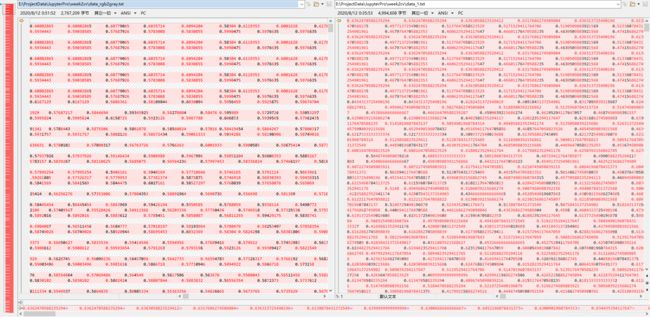虽然计算的结果不同但是二者都能输出灰度图, 且最终的呈现效果并无差异, 那么造成数据不一致的可能是计算的公式不一致吗 ?

查询资料发现 Gray = R*0.299 + G*0.587 + B*0.114 计算公式是由标准的RGB三元色通过Gamma矫正得到的.而skimage中的Gray值是通过RGB对当前CRT荧光屏校准得到的：Gray = 0.2125 * R + 0.7154 * G + 0.0721 * B , 如此, 我们需要换一种算法,再验证一次,看看效果.除了计算的精度差异之外,两组数据基本一致.

# Gray = float(0.2125 * R + 0.7154 * G + 0.0721 * B) / 255

import numpy as np

import matplotlib.pyplot as plt

from PIL import Image

import cv2

# 读取图片

# cv2.imshow("lenna_png", img)

# cv2.waitKey(0)

img_height, img_weight, channel = img.shape

dest = np.ndarray((img_height,img_weight))

for img_y in range(img_height):

for img_x in range(img_weight):

R = img[img_y, img_x, 2]

G = img[img_y, img_x, 1]

B = img[img_y, img_x, 0]

gray_value = float(0.2125 * R + 0.7154 * G + 0.0721 * B) / 255

# print(gray_value)

dest[img_y, img_x] = round(gray_value, 8)

# 将数据输出到txt文件中方便后面对比数据差异

fp = open("data_2.txt", mode = 'w')

for img_y in range(img_height):

for img_x in range(img_weight):

fp.write(str(dest[img_y, img_x]))

fp.write("\t")

fp.write("\n")

print(dest)

plt.imshow(dest, cmap = 'gray')

# cv2.waitKey()

plt.show()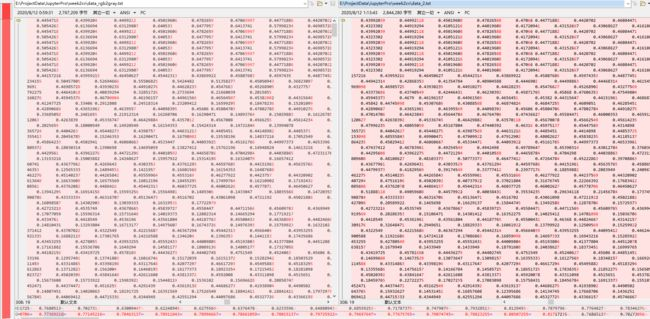灰度图也可以正常显示,总结来说,无论是哪一种公式实现的灰度图,其本质上都是将三通道的彩色数据均值化到一通道数据的映射,只要标准统一.其结果基本一致.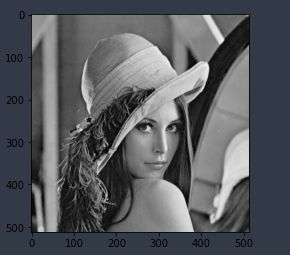展开全文•matlab
• 以R、G、B为轴建立空间直角坐标系，则RGB图的每个象素的颜色可以用该三维空间的一个点...于是rgbgray图的本质就是寻找 一个三维空间到一维空间的映射，最容易想到的就是射影（即过rgb空间的一个点向直线R=G=B做垂线）
• rgb2grayRGB 图像或颜色图转换为灰度图 语法 说明 示例 将 RGB 图像转换为灰度图像 将 RGB 颜色图转换为灰度颜色图 输入参数 输出参数 提示 算法 rgb2grayRGB 图像或颜色图转换为灰度图 语法 I =...

目录

语法

说明

示例

将 RGB 图像转换为灰度图像

将 RGB 颜色图转换为灰度颜色图

rgb2gray将 RGB 图像或颜色图转换为灰度图

## 语法

I = rgb2gray(RGB)

newmap = rgb2gray(map)

## 说明

I = rgb2gray(RGB) 将真彩色图像 RGB 转换为灰度图像 I。rgb2gray 函数通过消除色调和饱和度信息，同时保留亮度，来将 RGB 图像转换为灰度图。如果已安装 Parallel Computing Toolbox™，则 rgb2gray 可以在 GPU 上执行此转换。

newmap = rgb2gray(map) 返回等同于map的灰度颜色图。

## 示例

### 将 RGB 图像转换为灰度图像

读取并显示 RGB 图像，然后将其转换为灰度图。读取示例文件 peppers.png 并显示 RGB 图像。

RGB = imread('peppers.png');
imshow(RGB)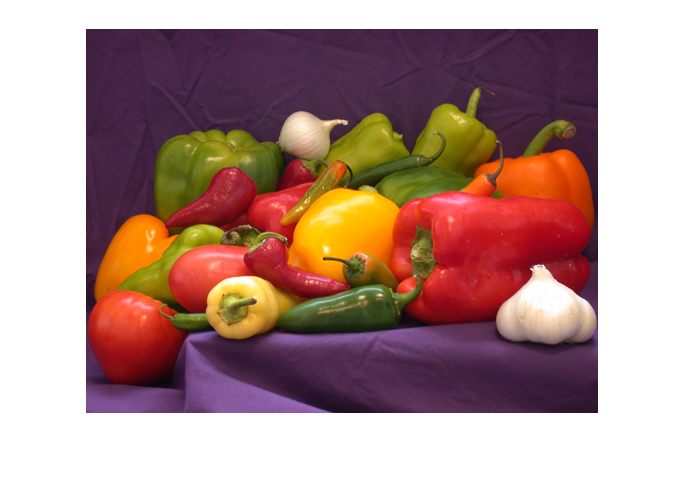将 RGB 图像转换为灰度图像并显示图像。

I = rgb2gray(RGB);
figure
imshow(I)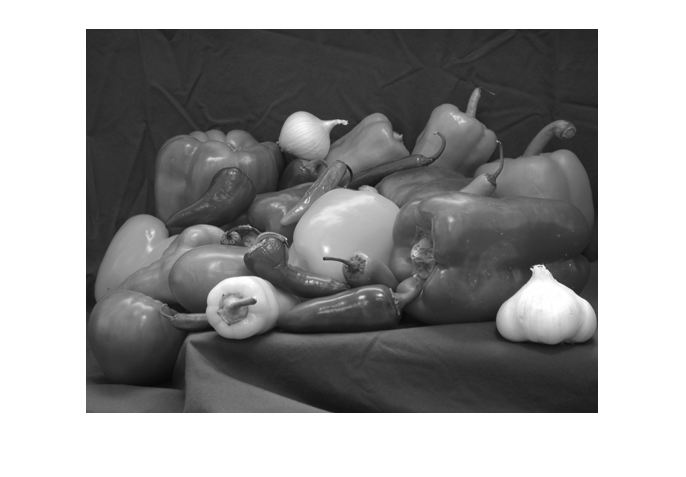### 将 RGB 颜色图转换为灰度颜色图

读取具有 RGB 颜色图的索引图像。然后将颜色图转换为灰度。读取示例文件 corn.tif，该文件为具有 RGB 颜色图的索引图像。

[X,map] = imread('corn.tif');

显示图像。

imshow(X,map)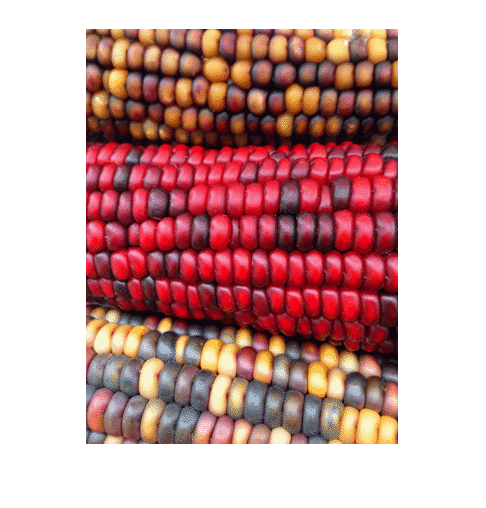将 RGB 颜色图转换为灰度颜色图并重新显示图像。

newmap = rgb2gray(map);
imshow(X,newmap)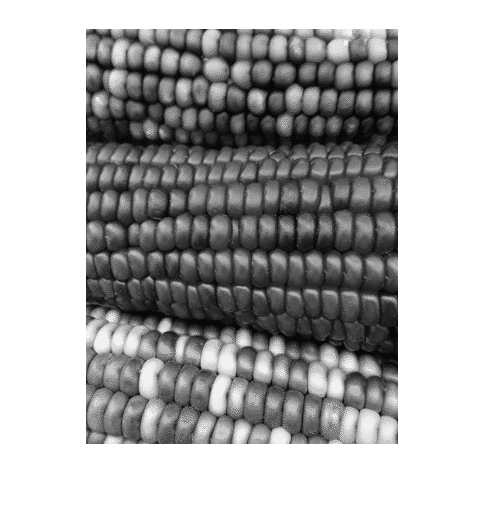RGB - 真彩色图像
m×n×3 数值数组

真彩色图像，指定为 m×n×3 数值数组。如果已安装 Parallel Computing Toolbox，则 RGB 也可以是 gpuArray。

map - 颜色图
c×3 数值矩阵

颜色图，指定为由范围 [0, 1] 内的值组成的 c×3 数值矩阵。map 的每行都是一个三元素 RGB，指定颜色图的单种颜色的红、绿和蓝分量。如果已安装 Parallel Computing Toolbox，则 map 也可以是 gpuArray。

I - 灰度图片
m×n 数值数组

灰度图像，以 m×n 数值数组形式返回。如果已安装 Parallel Computing Toolbox，则 I 也可以是 gpuArray。

newmap - 灰度颜色图
c×3 数值矩阵

灰度颜色图，返回为由范围 [0, 1] 内的值组成的 c×3 数值矩阵。newmap 的三列是相同的，因此map的每行都指定一个强度值。如果已安装 Parallel Computing Toolbox，则 newmap 也可以是 gpuArray。

提示

• rgb2gray 支持使用 MATLAB® Coder™ 生成 C 代码。

算法

rgb2gray 通过计算 R、G 和 B 分量的加权和，将 RGB 值转换为灰度值：

0.2989 * R + 0.5870 * G + 0.1140 * B 

这些权重与rgb2ntsc (Image Processing Toolbox) 函数用于计算 Y 分量的权重相同。在舍入到小数点后 3 位之后，rgb2gray中用来计算灰度值的系数与Rec.ITU-R BT.601-7 中用来计算亮度 (E'y) 的系数相同。

Rec.ITU-R BT.601-7 使用以下公式计算 E'y：

0.299 * R + 0.587 * G + 0.114 * B

展开全文matlab 计算机视觉 图像处理
• RGB文件转为灰度图后，生成灰度图的相应数据，并保存为.coe文件，供rom初始化时使用。
• ## matlab rgb2gray() 的坑

千次阅读 2022-01-08 20:14:35
ima = double(rgb2gray(uint8(ima))); 特别注意在在使用rgb2gray这个函数将3通道转化成单通道的时候，必须先将这个图像转化成uint8类型。如果不进行转化的话，由于此处的ima在读取之后变成了double数据类型，直接...
ima = double(imread('data/house.png'));
ima = double(rgb2gray(uint8(ima)));


特别注意在在使用rgb2gray这个函数将3通道转化成单通道的时候，必须先将这个图像转化成uint8类型。如果不进行转化的话，由于此处的ima在读取之后变成了double数据类型，直接使用rgb2gray(ima)得到的矩阵的取值范围是在0-1范围之内的！这与造成imshow显示图像为全白的原因是一样的，imshow函数和rgb2gray函数，都会将double类型的数据视作0-1数值范围内的，如果原数据中含有大于1的数值，则这些数值都会被改为1！而imshow和rgb2gray函数把uint8类型的数据视作0-255范围之内的！

参考Imshow https://blog.csdn.net/tm807782912/article/details/46879315

展开全文matlab 计算机视觉 opencv
• saperate rgb planes.rar,saperate rgb planes.docx
• 我正在尝试对一个图像执行直方图均衡化有两个问题。...我的代码如下：img = cv2.imread(r'D:/UNI/Y3/DIA/2K18/lab.jpg')RGB_img = cv2.cvtColor(img, cv2.COLOR_BGR2RGB)gray = cv2.cvtColor(RGB_img,...
• convert rgb to grayscale

千次阅读 热门讨论 2020-05-29 14:36:13
通过以下公式可以将RGB转换成灰度 GRAY＝0.2989*R + 0.5870*G+ 0.1140*B 在Matlab中为我们提供了rgb2gray函数，将 RGB 图像或颜色图转换为灰度图，用法为： I = rgb2gray(RGB) newmap = rgb2gray(map) I = rgb2gray...
• cvtColor(src, grayImg, CV_RGB2GRAY); 则会出现报错，是因为opencv更新后的版本问题 解决方法1： 将上述代码改为： cvtColor(src, grayImg, COLOR_RGB2GRAY); 解决方法2： 加入头文件： #include <opencv2\...
• code for converting gray scale image into color image by using templatescale
• 平时处理图像的时候，很少用到彩色信息，一般...转换灰度图用的cvtColor的第三个参数中有两个参数，我一直没太注意区别，CV_RGB2GRAY与CV_BGR2GRAY。今天通过一个简单的测试区分一下。 测试代码如下： #include ...OpenCV
• matlab开发-rgb2gray。不同方法转换rgb2gray的比较
• ## matlab rgb2gray的实现

万次阅读 2018-11-12 12:10:13
rgb2gray是matlab内部一种处理图像的函数，通过消除图像色调和饱和度信息同时保留亮度实现将将RGB图像或彩色图转换为灰度图像，即灰度化处理的功能，调用这个功能的格式是I = rgb2gray(RGB)，意思是将真彩色图像RGB...
• 使用 Vivado HLS 实现一个图像处理的 IP 核，该 IP 核能利用 xfopencv 将 OV5640 摄像头产生的 RGB 彩色图像转换成灰度图像，在 Vivado 中对 IP 核进行验证。最后通过 HDMI 实时显示。 工具 1.Vivado HLS 2019.1 2....opencv fpga开发
• ## 图像处理：rgb2gray

千次阅读 2019-06-13 10:04:25
这个函数比较简单，matlab 网站上给出的大约是这个样子， 0.2989R+ 0.5870G + 0.1140B 注意，如果程序中直接写成浮点乘法，相对比较消耗时间，一般我会写成整数...gray = (R*77 + G*150 + B*29) >> 8; ...
• 出现这种情况是你使用的图像是因为是8位色深度的图片，可以使用qq截图等方式将其截图下来改为24位色深度的图片就可以解决其问题。 参考了网上的网友的说法就解决了该问题的。感谢 ......
• Matlab文件夹批量操作文件实例-new_rgb2gray.m 看了帖子“https://www.ilovematlab.cn/thread-46154-1-1.html”首先感谢goodlucklars 对文件夹操作有了新的认识，结合以前hehaihuiwang的一个gui代码中对文件夹...matlab
• ## OpenCV CV_RGB2GRAY与CV_BGR2GRAY的区别

万次阅读 多人点赞 2018-06-25 17:03:10
转换灰度图用的cvtColor的第三个参数中有两个参数，我一直没太注意区别，CV_RGB2GRAY与CV_BGR2GRAY。今天通过一个简单的测试区分一下。测试代码如下：#include &lt;opencv2\opencv.hpp&gt; using namespace......